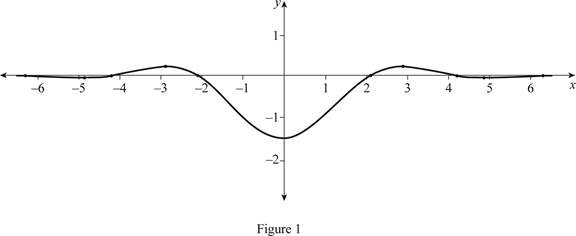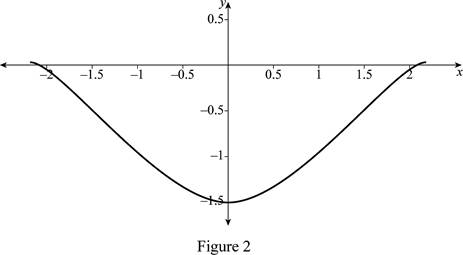# The value of lim x → 0 cos 2 x − cos x x 2 by graphing and find where it crosses the y -axis.### Single Variable Calculus: Concepts...

4th Edition
James Stewart
Publisher: Cengage Learning
ISBN: 9781337687805### Single Variable Calculus: Concepts...

4th Edition
James Stewart
Publisher: Cengage Learning
ISBN: 9781337687805

#### Solutions

Chapter 2.2, Problem 25E

(a)

To determine

## The value of limx→0cos2x−cosxx2 by graphing and find where it crosses the y-axis.

Expert Solution

The value of limx0cos2xcosxx2=1.5.

### Explanation of Solution

Using the online graphing calculator and draw the graph of the function f(x)=cos2xcosxx2 as shown below in Figure 1.Zoom in the graph towards the point where the graph crosses the y-axis as shown below in Figure 2.From Figure 2, it is observed that graph of f(x)=cos2xcosxx2 approaches −1.5 as x approaches 0 from either side.

That is, limx0cos2xcosxx2=limx0+cos2xcosxx2=1.5.

Since the right hand limits and the left hand limits are equal, the value of limx0cos2xcosxx2 exists.

Thus, the value of limx0cos2xcosxx2=1.5.

(b)

To determine

### The answer obtained in part (a) by evaluating the value of cos2x−cosxx2 as x approaches 0.

Expert Solution

The value of limx0cos2xcosxx2=1.5.

### Explanation of Solution

As x approaches 0, evaluate the function f(x)=cos2xcosxx2 for the numbers 0.5,0.1,0.01,0.001,0.0001,0.5,0.1,0.01,0.001 and 0.0001.

Evaluate the function (correct to 6 decimal places) for the values of x and get the following table of values.

 x cos2x−cosx x2 f(x)=cos2x−cosxx2 −0.5 −0.337280 0.25 −1.349121 −0.1 −0.014937 0.01 −1.493758 −0.01 −0.000149 0.0001 −1.499937 −0.001 −0.0000015 0.000001 −1.499999 −0.0001 −0.000000015 0.00000001 −1.499999 0.5 −0.337280 0.25 −1.349121 0.1 −0.014937 0.01 −1.493758 0.01 −0.000149 0.0001 −1.499937 0.001 −0.0000015 0.000001 −1.499999 0.0001 −0.000000015 0.00000001 −1.499999

Here, it is observed that the value of f(x) gets closer to −1.5 as x approaches to 0 from the either side. That is, limx0cos2xcosxx2=1.5.

Therefore, it is verified from the table that limx0cos2xcosxx2=1.5.

### Have a homework question?

Subscribe to bartleby learn! Ask subject matter experts 30 homework questions each month. Plus, you’ll have access to millions of step-by-step textbook answers!